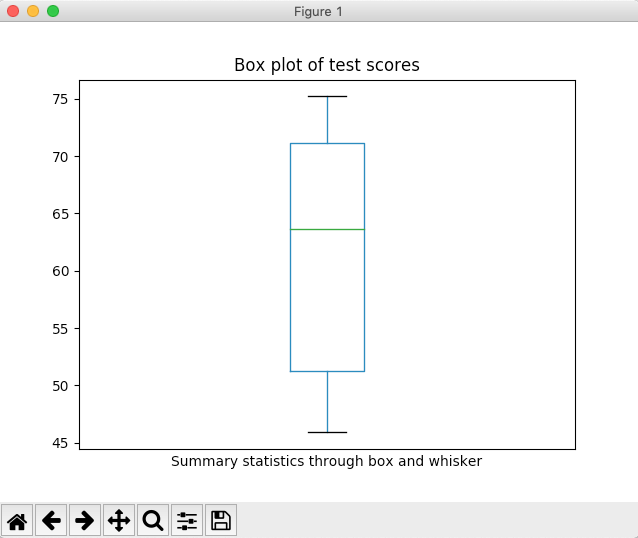# Drawing A Box Plot Using Pandas Series

## Overview:

• A box and whisker plot or simply box plot draws a box, using the 25th percentile and 75th percentile of the distribution as its boundaries.
• Median value is marked in the box.
• The box is marked with whiskers that extend up to the minimum value and the maximum value of the distribution.
• Outliers are marked as bubbles.

## Drawing a Box plot for the data present in a pandas.Series instance:

• The box() function can be called on the plot member of the Series instance, which plots the box plot for the data.
• In the same way, to draw box plot for a pandas DataFrame the DataFrame.plot.box() function can be used.

## Example:

 # Example Python program to draw a # box-whisker plot for the data present in a pandas Series import pandas as pds import matplotlib.pyplot as plt   # Test scores testScores  = [75.2, 45.9, 52, 51, 60, 71, 46.4, 67.2, 74, 71.2];   # Load test scores into a pandas Series series      = pds.Series(testScores); series.plot.box(title="Box plot of test scores", xticks=[]);   # Print the summary statistics print(series.describe());   plt.xlabel('Summary statistics through box and whisker'); plt.show(block=True);

## Output:

 count    10.000000 mean     61.390000 std      11.713093 min      45.900000 25%      51.250000 50%      63.600000 75%      71.150000 max      75.200000 dtype: float64